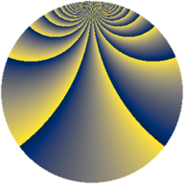# Properties

 Label 2300.2.btLevel $2300$ Weight $2$ Character orbit 2300.bt Rep. character $\chi_{2300}(3,\cdot)$ Character field $\Q(\zeta_{220})$ Dimension $28480$ Sturm bound $720$

# Related objects

## Defining parameters

 Level: $$N$$ $$=$$ $$2300 = 2^{2} \cdot 5^{2} \cdot 23$$ Weight: $$k$$ $$=$$ $$2$$ Character orbit: $$[\chi]$$ $$=$$ 2300.bt (of order $$220$$ and degree $$80$$) Character conductor: $$\operatorname{cond}(\chi)$$ $$=$$ $$2300$$ Character field: $$\Q(\zeta_{220})$$ Sturm bound: $$720$$

## Dimensions

The following table gives the dimensions of various subspaces of $$M_{2}(2300, [\chi])$$.

Total New Old
Modular forms 29120 29120 0
Cusp forms 28480 28480 0
Eisenstein series 640 640 0

## Trace form

 $$28480q - 72q^{2} - 90q^{4} - 144q^{5} - 54q^{6} - 72q^{8} - 180q^{9} + O(q^{10})$$ $$28480q - 72q^{2} - 90q^{4} - 144q^{5} - 54q^{6} - 72q^{8} - 180q^{9} - 72q^{10} - 84q^{12} - 144q^{13} - 90q^{14} - 54q^{16} - 144q^{17} - 52q^{18} - 76q^{20} - 108q^{21} - 172q^{22} - 144q^{25} - 144q^{26} - 56q^{28} - 180q^{29} - 92q^{30} - 92q^{32} - 120q^{33} - 90q^{34} - 22q^{36} - 144q^{37} - 80q^{38} - 192q^{40} - 108q^{41} + 112q^{42} - 90q^{44} - 280q^{45} - 60q^{46} - 300q^{48} - 236q^{50} - 20q^{52} - 96q^{53} - 350q^{54} - 54q^{56} - 136q^{57} - 32q^{58} - 48q^{60} - 108q^{61} - 108q^{62} - 90q^{64} - 112q^{65} - 6q^{66} - 196q^{68} - 200q^{69} - 216q^{70} - 256q^{72} - 144q^{73} - 176q^{76} - 136q^{77} - 100q^{78} - 294q^{80} - 756q^{81} - 80q^{82} + 70q^{84} + 72q^{85} - 54q^{86} - 40q^{88} - 180q^{89} - 354q^{90} + 22q^{92} - 472q^{93} - 90q^{94} - 94q^{96} - 144q^{97} - 20q^{98} + O(q^{100})$$

## Decomposition of $$S_{2}^{\mathrm{new}}(2300, [\chi])$$ into newform subspaces

The newforms in this space have not yet been added to the LMFDB.